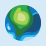# Script to turn errors into no data (Desktop 10.3)

2186
13
01-15-2016 10:27 AMOccasional Contributor

I am running a field calculation which is [field A]/[field B].  Some of the values in field B are zero.  Is there a script I could use to turn results in the calculation where the denominator is zero into -99 instead of creating an error message?

Tags (4)
1 Solution

Accepted SolutionsbyMVP Honored Contributor

Put this in the code block:

```def my_function(numerator, denominator):
if denominator == 0:
return -99
else:
return numerator/denominator ```

...and this in the expression below the code block:

`my_function(!CHSCH04!,!TOSCH04!)`

Make sure you've changed to the Python parser.

13 RepliesbyMVP Honored Contributor

Something like:

Codeblock:

```def my_function(numerator, denominator):
if denominator == 0:
denominator = -99
return numerator/denominator```

Expression:

`my_function( !YOUR_NUMERATOR_FIELD!, !YOUR_DENOMINATOR_FIELD! )`byMVP Esteemed Contributor

Maybe the result can return just the value of -99 instead of numerator/-99 if the denominator is 0. Rest seems perfect.

Think LocationbyMVP Honored Contributor

Oh, sure, yes I missed that part:

```def my_function(numerator, denominator):
if denominator == 0:
return -99

else:
return numerator/denominator```Occasional Contributor

Maybe I am messing up the syntax.  Here is what I have:

Codeblock:

def my_function(numerator, denominator):

if denominator == 0:

return -99

else:

return numerator/denominator

PRCHSCH =

my_function( [CHSCH04] , [TOSCH04] )

Still returning an error.   Thanks for the help.byMVP Honored Contributor

Should have mentioned you need to use the Python parser with this example, although to be fair you did tag Python. Field names should be enclosed in exclamation marks.Occasional Contributor

Darren -

I should have specified my level of experience with python.  The formula makes sense but I am still messing up the syntax and I have tried it several ways.  My fields are titled CHSCH04, which is the numerator, and TOSCH04, which is the denominator.  Can you please tell me exactly how to put those in your script and in the formula?  Thanks for your help.

StevebyMVP Honored Contributor

Put this in the code block:

```def my_function(numerator, denominator):
if denominator == 0:
return -99
else:
return numerator/denominator ```

...and this in the expression below the code block:

`my_function(!CHSCH04!,!TOSCH04!)`

Make sure you've changed to the Python parser.Occasional Contributor

That was my problem.  I did not change to python.

Now there are no errors and those with no value in the denominator are coded as -99.  The calculation returns a value of only 1 or 0 though.  It is not rounding as only those values that should equal 1 are returning that.  Every other value below 1 returns a 0.  Additionally, the field is a float set to 6 decimal places.

Any ideas?Occasional Contributor

Additionally, when I switch back to VB and select a feature with values of 7 for CHSCH04 and 13 for TOSCH04 and enter the formula [CHSCH04] / [TOSCH04] it returns the correct value of 0.538462.